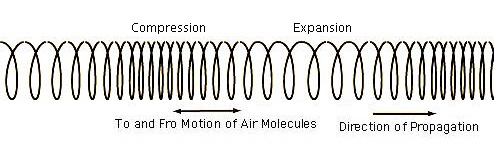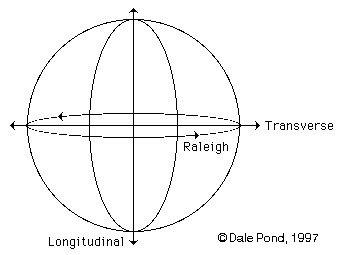Figure 8.3 - Coiled Spring showing Longitudinal WaveFigure 8.3 - Coiled Spring showing Longitudinal Wave

Longitudinal Axis
Triple axis of the three modes of vibration drawn to reference a circle and sphere.

The three modes working together develop rotation by and through each other's motion - the rotation is the Raleigh Wave or Surface Wave mode. The first two modes cause (or are) straight line and zig-zag motions only. The third or Raleigh Wave is circular.Longitudinal Axis

Longitudinal Waves, Velocity of

8.3 - Conventional View of Wave Motion
Compression Wave
Compression Wave Velocity
Figure 8.2 - Compression Wave Phase Illustration
Figure 9.11 - Compression Wave with expanded and contracted Orbits
Longitudinal Wave
Modes of Vibration
Sine Wave
Sound
Wave
Wave Field